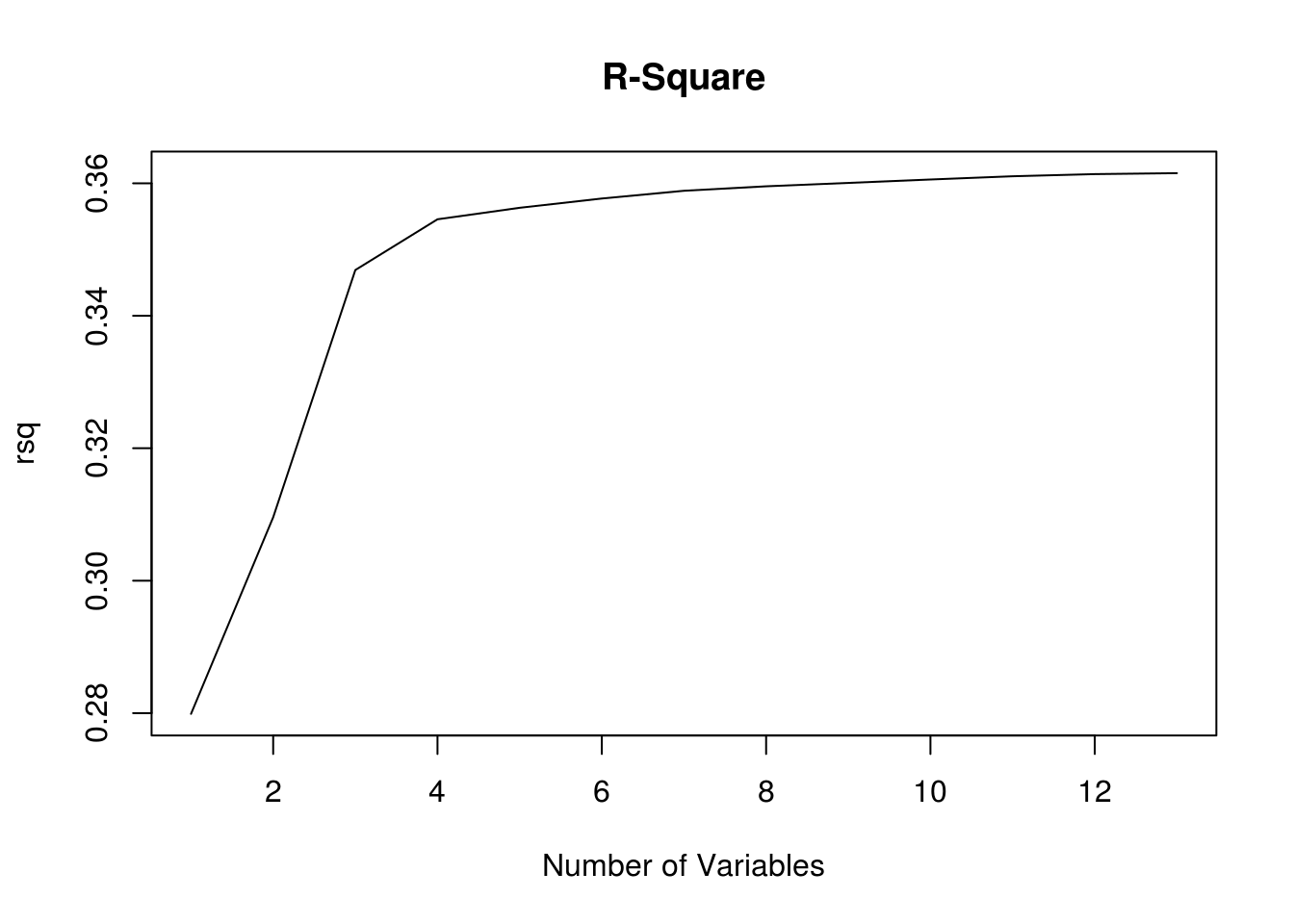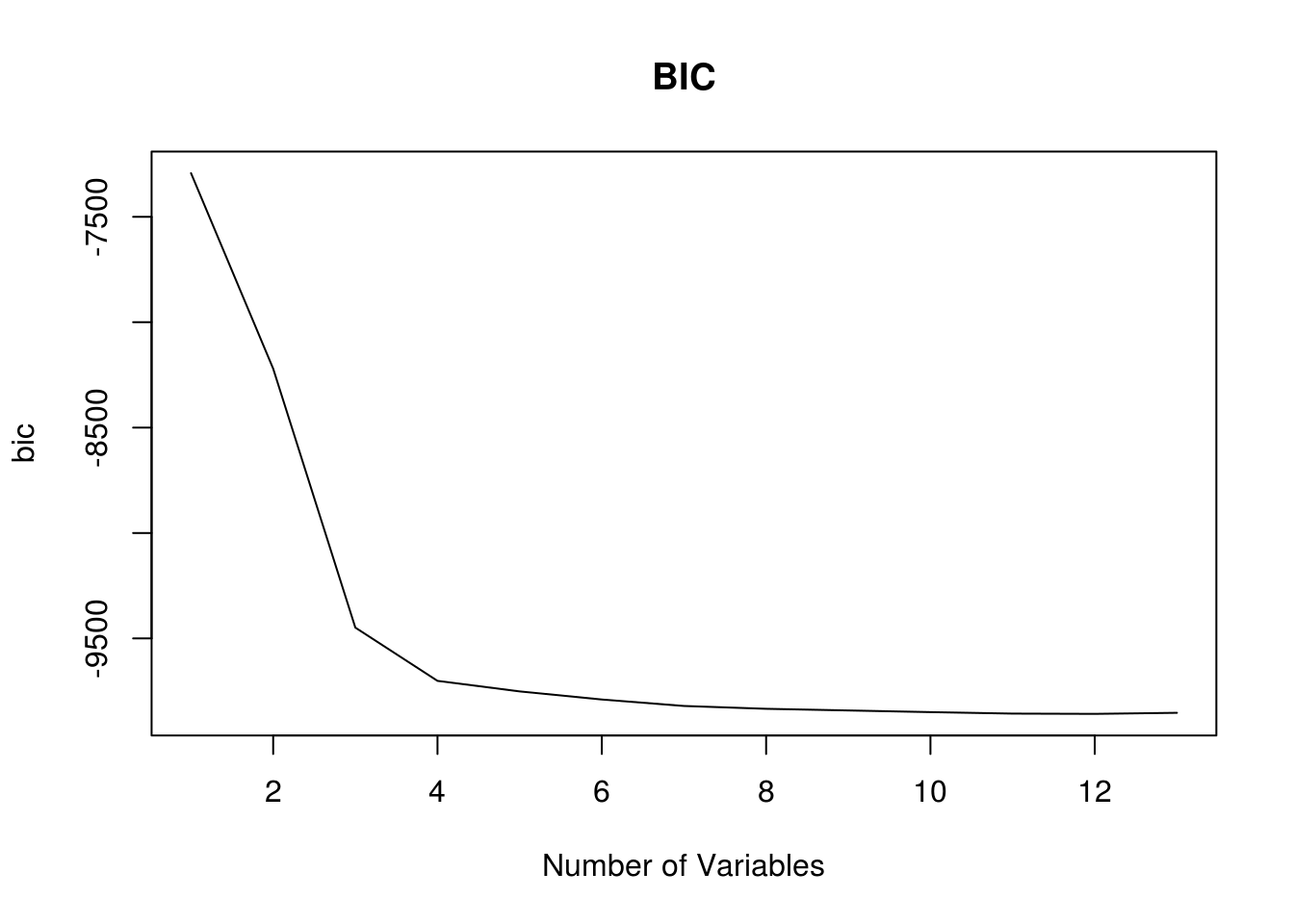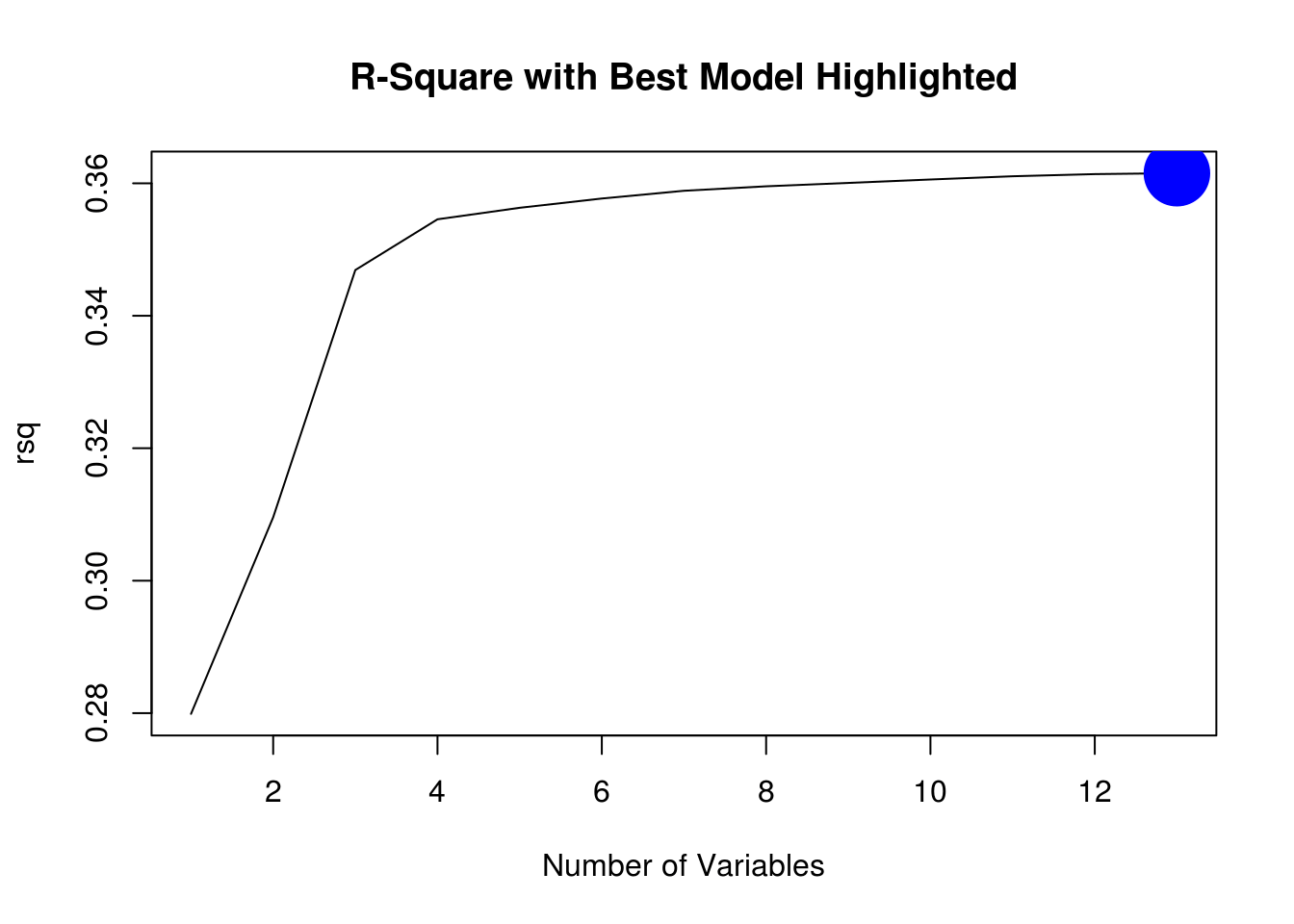# Example of Best Subset Regression in R

This post will provide an example of best subset regression. This is a topicthat has been covered before in this blog. However, in the current post, we will approach this using a slightly different coding and a different dataset. We will be using the “HI” dataset from the “Ecdat” package. Our goal will be to predict the number of hours a women works based on the other variables in the dataset. Below is some initial code.

``library(leaps);library(Ecdat)``
``````data(HI)
str(HI)``````
``````## 'data.frame':    22272 obs. of  13 variables:
##  \$ whrswk    : int  0 50 40 40 0 40 40 25 45 30 ...
##  \$ hhi       : Factor w/ 2 levels "no","yes": 1 1 2 1 2 2 2 1 1 1 ...
##  \$ whi       : Factor w/ 2 levels "no","yes": 1 2 1 2 1 2 1 1 2 1 ...
##  \$ hhi2      : Factor w/ 2 levels "no","yes": 1 1 2 2 2 2 2 1 1 2 ...
##  \$ education : Ord.factor w/ 6 levels "<9years"<"9-11years"<..: 4 4 3 4 2 3 5 3 5 4 ...
##  \$ race      : Factor w/ 3 levels "white","black",..: 1 1 1 1 1 1 1 1 1 1 ...
##  \$ hispanic  : Factor w/ 2 levels "no","yes": 1 1 1 1 1 1 1 1 1 1 ...
##  \$ experience: num  13 24 43 17 44.5 32 14 1 4 7 ...
##  \$ kidslt6   : int  2 0 0 0 0 0 0 1 0 1 ...
##  \$ kids618   : int  1 1 0 1 0 0 0 0 0 0 ...
##  \$ husby     : num  12 1.2 31.3 9 0 ...
##  \$ region    : Factor w/ 4 levels "other","northcentral",..: 2 2 2 2 2 2 2 2 2 2 ...
##  \$ wght      : int  214986 210119 219955 210317 219955 208148 213615 181960 214874 214874 ...``````

To develop a model we use the “regsubset” function from the “leap” package. Most of the coding is the same as linear regression. The only difference is the “nvmax” argument which is set to 13. The default setting for “nvmax” is 8. This is good if you only have 8 variables. However, the results from the “str” function indicate that we have 13 functions. Therefore, we need to set the “nvmax” argument to 13 instead of the default value of 8 in order to be sure to include all variables. Below is the code

``regfit.full<-regsubsets(whrswk~.,HI, nvmax = 13)``

We can look at the results with the “summary” function. For space reasons, the code is shown but the results will not be shown here.

``summary(regfit.full)``

If you run the code above in your computer you will 13 columns that are named after the variables created. A star in a column means that that variable is included in the model. To the left is the numbers 1-13 which. One means one variable in the model two means two variables in the model etc.

Our next step is to determine which of these models is the best. First, we need to decide what our criteria for inclusion will be. Below is a list of available fit indices.

``names(summary(regfit.full))``
``##  "which"  "rsq"    "rss"    "adjr2"  "cp"     "bic"    "outmat" "obj"``

For our purposes, we will use “rsq” (r-square) and “bic” “Bayesian Information Criteria.” In the code below we are going to save the values for these two fit indices in their own objects.

``````rsq<-summary(regfit.full)\$rsq
bic<-summary(regfit.full)\$bic``````

Now let’s plot them

``plot(rsq,type='l',main="R-Square",xlab="Number of Variables")````plot(bic,type='l',main="BIC",xlab="Number of Variables")``You can see that for r-square the values increase and for BIC the values decrease. We will now make both of these plots again but we will have r tell the optimal number of variables when considering each model index. For we use the “which” function to determine the max r-square and the minimum BIC

``which.max(rsq)``
``##  13``
``which.min(bic)``
``##  12``

The model with the best r-square is the one with 13 variables. This makes sense as r-square always improves as you add variables. Since this is a demonstration we will not correct for this. For BIC the lowest values was for 12 variables. We will now plot this information and highlight the best model in the plot using the “points” function, which allows you to emphasis one point in a graph

``````plot(rsq,type='l',main="R-Square with Best Model Highlighted",xlab="Number of Variables")
points(13,(rsq),col="blue",cex=7,pch=20)````````````plot(bic,type='l',main="BIC with Best Model Highlighted",xlab="Number of Variables")
points(12,(bic),col="blue",cex=7,pch=20)``````Since BIC calls for only 12 variables it is simpler than the r-square recommendation of 13. Therefore, we will fit our final model using the BIC recommendation of 12. Below is the code.

``coef(regfit.full,12)``
``````##        (Intercept)             hhiyes             whiyes
##        30.31321796         1.16940604        18.25380263
##        education.L        education^4        education^5
##         6.63847641         1.54324869        -0.77783663
##          raceblack        hispanicyes         experience
##         3.06580207        -1.33731802        -0.41883100
##            kidslt6            kids618              husby
##        -6.02251640        -0.82955827        -0.02129349
## regionnorthcentral
##         0.94042820``````

So here is our final model. This is what we would use for our test set.

Conclusion

Best subset regression provides the researcher with insights into every possible model as well as clues as to which model is at least statistically superior. This knowledge can be used for developing models for data science applications.